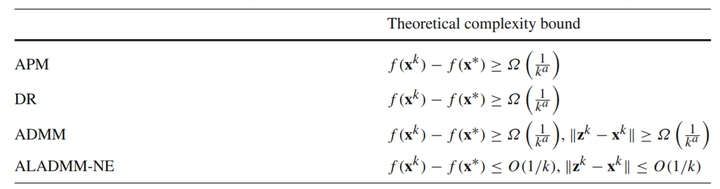# Accelerated Alternating Direction Method of Multipliers:An Optimal O(1/K) Nonergodic Analysis### Abstract

The Alternating Direction Method of Multipliers (ADMM) is widely used for linearly constrained convex problems. It is proven to have an o(1/√K) nonergodic convergence rate and a faster O(1/K) ergodic rate after ergodic averaging, where K is the number of iterations. Such nonergodic convergence rate is not optimal. Moreover, the ergodic averaging may destroy the sparseness and low-rankness in sparse and low-rank learning. In this paper, we modify the accelerated ADMM proposed in Ouyang et al. (SIAM J. Imaging Sci. 7(3):1588–1623, 2015) and give an O(1/K) nonergodic convergence rate analysis, which satisfies |F(xK ) − F(x∗)| ≤ O(1/K), ||AxK − b|| ≤ O(1/K) and xK has a more favorable sparseness and low-rankness than the ergodic peer, where F(x)is the objective function and Ax = b is the linear constraint. As far as we know, this is the first O(1/K) nonergodic convergent ADMM type method for the general linearly constrained convex problems. Moreover, we show that the lower complexity bound of ADMM type methods for the separable linearly constrained nonsmooth convex problems is O(1/K), which means that our method is optimal.

Type
Publication
Journal of Scientific Computing